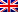#[Sharif University CTF 2016] [Misc 300 – Sec-Coding 2] Write Up

## Description

You should fix vulnerabilities of the given source code, WITHOUT changing its normal behaviour.

Rules & Notes

MISSION: You should fix vulnerabilities of the given source code, WITHOUT changing its normal behaviour.
RULE0: When an honest user gives a non-malicious (but maybe incorrect) input which does not trigger the vulnerabilities,
the output of uploaded fixed code should be the same as before.
RULE1: When the attacker gives his/her attack vector, your program should not crash or do dangerous actions (explained below),
but continue its execution and exit normally at the end. In this situation, your program is allowed to output anything.
RULE2: A (poorly-tested) source code may crash even when interacting with a normal user. You should fix these cases too.
(NOTE: the output should be correct in this case)
Dangerous actions (stated above) includes buffer overflows, writing to unallocated memory address, reading uninitialized memory,
and any other programming mistakes leading to crash/instability.
Some prevention techniques, detect the attack and prevent memory corruption but throw an exception which terminates the program,
leading to denial of service. You should avoid such termination and the program should recover from the attack, continue execution,
and exit normally at the end.

```#include <math.h>
#include <stdio.h>
#include <windows.h>

int main(int argc, char **argv)
{
// STRING ECHO
//
// Sample usage:

char *str = (char *)malloc(100);
int repeat = 0;

char *line = GetCommandLineA();

while (*line != ' ')
line++;
line++;

if (strncmp(line, "repeat=", 7) == 0)
{
line += 7;
repeat = atoi(line);
line += (int)ceil(log10((double)repeat)) + 1;
}

if (strncmp(line, "str=", 4) == 0)
{
line += 4;
str = strtok(line, " ");
}

for (int i = 0; i < repeat; i++)
printf("%s\n", str);

line += strlen(str);
for (; line >= GetCommandLineA(); line--)
*line = '\x0';

free(str);

return -14;
}
```

## Resolution

This was a little bit harder than the first one. Basically we had to patch every function that could fail and be sure to output something when an attack vector was detected.
strtok can return NULL if no token is found, so we quit in that case, we also need to check if the program is launched without any argument and then exit.
And lastly, we need to change the calculation of the repeat length number which could overflow or have a negative value.
Our final code is here:

```#include <math.h>
#include <stdio.h>
#include <windows.h>

int main(int argc, char **argv)
{
// STRING ECHO
//
// Sample usage:

char *str;
int repeat = 0;

char *line = GetCommandLineA();

while (*line != '\x0' && *line != ' ')
line++;
if (*line == '\x0')
{
printf("Malicious\n");
return -14;
}
line++;

if (strncmp(line, "repeat=", 7) == 0)
{
line += 7;
repeat = atoi(line);
if (repeat <= 0)
{
printf("Malicious\n");
return -14;
}
line += (int)floor(log10((double)abs(repeat))) + 1;
}
line++;
if (strncmp(line, "str=", 4) == 0)
{
line += 4;
str = strtok(line, " ");
if (str == NULL)
{
printf("Malicious\n");
return -14;
}
}

for (int i = 0; i < repeat; i++)
printf("%s\n", str);

line += strlen(str);
for (; line >= GetCommandLineA(); line--)
*line = '\x0';

return 0;
}
```

The flag is: 983027cec2889d128529c078eebb6471